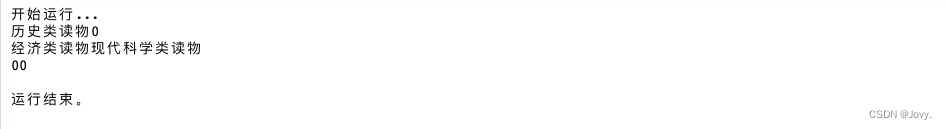0

# 【Java数组】一维数组与二维数组（附题目）## 数组表示方法

``````public class Arr{
public static void main(String[] args){
int[] a;                先声明数据类型 type[] 标识符；
再实例化这个数组 标识符=new type[列表长度]
a = new int;
}
}
``````
``````public class Arr{
public static void main(String[] args){
int[] a = new int;        //type[] 标识符 = new type[列表长度]
int a[] = new int;      //type 标识符[] = new type[列表长度]
//上下两种均可，推荐用上面那个！
}
}
``````

## 一维数组初始化

``````public class Arr{
public static void main(String[] args){
int[] a;
a = new int;
int[] a = {1,2,3}        //type[] 标识符 = {元素}
}
}
``````
``````public class Arr{
public static void main(String[] args){
int[] a = new int[]{元素};        //这里可以根据元素个数决定元素长度

}
}
``````

## 二维数组

``````public class Arr{
public static void main(String[] args){
int[][] a = new int;
}
}
``````

## 题目

``````public class Arr {
public static void main(String[] args){

String book = "历史类读物";        //创建一个字符串
String book1 = "经济类读物";
String book2 = "现代科学类读物";
String[][] cabinet ={{book,"0"},{book1,book2},{"0","0"}};    //创建二维数组
for(int i=0;i<cabinet.length;i++){    //套用for循环，因为要有三层，所以有三次循环
for(int j=0;j<cabinet.length;j++){    //for循环，因为有两列，所以循环两次
System.out.print(cabinet[i][j]);    //因为ij表示的分别是次数，也可以在列表中表示索引，i最大是2，j最大是1
}
System.out.println();    //输出空格
}

}

}
``````## 末尾抽福袋活动！

### “【Java数组】一维数组与二维数组（附题目）”的评论:

##### 关于作者##### overfit同步小助手